# Problem Set 3: Functional Data Structures and Algorithms

### Due Thursday, February 28, 23:59

You are not allowed to use side effects in this problem set.

Version: 4

• Removed `SplayHeap.size` reference. You don't have to implement it.
Version: 3
• Runtime Requirements for SplayHeap operations specified.
• Example input/output sequences added for T9 operations
• Added runtime specification for `size` function in the Splay Heap

1. Compile errors: All code you submit must compile. Programs that do not compile will probably receive an automatic zero. If you are having trouble getting your assignment to compile, please visit consulting hours. If you run out of time, it is better to comment out the parts that do not compile, than hand in a more complete file that does not compile.
2. Naming: We will be using an automatic grading script, so it is crucial that you name your functions and order their arguments according to the problem set instructions, and that you place the functions in the correct files. Otherwise you may not receive credit for a function properly written.
3. Code style: Finally, please pay attention to style. Refer to the CS 3110 style guide and lecture notes. Ugly code that is functionally correct may still lose points. Take the extra time to think out the problems and find the most elegant solutions before coding them up. Even though only the second part of the assignment explicitly addresses the style issue, good programming style is also required for the other parts of this assignment, and for all the subsequent assignments in the rest of the semester.
4. Late assignments: Please carefully review the course website's policy on late assignments, as all assignments handed in after the deadline will be considered late. Verify on CMS that you have submitted the correct version, before the deadline. Submitting the incorrect version before the deadline and realizing that you have done so after the deadline will be counted as a late submission.

## Part 1: Induction and The Substitution Model (30 pts)

One of the nice things about functional programming languages is the ease of reasoning about the programs we write. Some languages such as Coq have type systems and semantics powerful enough to essentially encode correctness proofs in the mere fact that a program compiles! Unfortunately, OCaml isn't quite there , but the goal of this exercise is to help give meaning to the age-old CS 3110 expression "If your code compiles, it probably works".

The substitution model of computation gives us a valuable fomalization and framework to start proving the correctness of programs. Our main proof technique will be induction. Induction is one of most fundamental and useful tools in proving statements about software. These exercises are meant to give you an idea of how widely applicable the induction principle is and to get you comfortable with reasoning about the correctness of your code. In each of the following exercises you should

1. Write a program to do the desired computation.
2. Prove that the program you wrote is correct.
You are highly encouraged to write your proofs in LaTeX* to generate a clean looking `.pdf`.

1. (5 points) Consider the following type definition for a data structure similar to the built in `List` module:
` type 'a list = Cons of 'a * 'a list | Zardoz`
Write a function `map : ('a -> 'b) -> 'a list -> 'b list` that behaves in an analogous manner to `List.map` and prove that your program is correct.
2. (10 points) This time we are going to take a slightly different spin on induction than before. Consider the following type definition for a functional tree:
`type tree = Node of int * tree * tree | Leaf`
Write a function `sum : tree -> int` that adds all of the integers contained in a `Node` element in the tree.

(Hint: For the proof, consider what happens when you reach a `Node` element in the tree traversal. What can you say about the number of subproblems left to solve? How can you use this to show that the program must terminate? Show that at termination, the program returns the correct value. This is a basic example of structural induction.)

3. (15 points) Now let's take this technique of proof a little bit further than we did in the previous two examples. We are going to study a special case of a tiling problem. We want to cover an n x n board with pieces called polyominoes, these are generalizations of just covering a board with dominoes, such that every square in the board is covered, but no two pieces intersect. A tiling that satisfies this condition is said to be valid. In general, finding a tiling is NP-complete, so the problem is hard... really hard. But we will consider a special case that has a known fast solution.

We consider a special type of polyomino called a L-shaped tromino. We will try to cover a 2^n x 2^n board with trominoes. The type abstractions will be given to you in the file `induction.ml`. Here is a visual representation of what each tromino orientation looks like: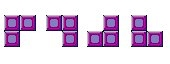Observe that the normal definition of valid cannot be satisfied by just these L-shaped trominos (the n = 1 case is a simple counterexample). We will need to modify our definition of valid. In this special case, a tiling is said to be valid if every square but the upper-left corner is covered.

1. Write a function ```find_tiling : int -> tiling``` that finds a valid tiling in a 2^n x 2^n board.
2. Write a proof by induction that your function returns a valid tiling. Note that this means you must verify the following things:
• The tiling that your function returns is valid.
• The tiling that your function returns covers all squares in the board (except the upper-left corner).
You may assume that n >= 1

We have provided you with a bash script to visualize the tiling if you want. Use the following to run it:

\$ ./print_tiling.sh

To submit: induction.ml, proofs.pdf.
*Using Windows? You'll want MiKTEX.

## Part 2: Splay Trees and the Functor Abstraction (25 points)

Until now, when working with data structures such as lists or trees, the underlying implementation of the data type was visible to us. For example, in Part 1 of this problem set we could reference the and `Node` elements of the `list` and `tree` data types, respectively. However, in practice, it is often not a great idea to let a client of a library reference the underlying representation. If the client code depends on the implementation of the libraries, then a change in the external library would break all of the client code! We can remedy this situation by using the module abstraction.

So what is a module? In essence, its an organized collection of more primitive objects that we have been working with the whole semester: types and functions involving those types. In OCaml, there is a distinction between a `module` and a `module type`. A `module type` is similar to an interface that you may be familiar with in object oriented programming: it is a description of the types and functions that the module will allow client code to see, and consequently every module of that module type satisfies this description.

We will want a general object that implements the `HEAP` signature. In essence, rather than passing a comparison function, we simply note that we could easily create a `HEAP` from anything that admits a comparison function (a totally ordered type). In essence, we can parameterize the `HEAP` module by any module that implements the `ORDERED`. The way to do this in functional programming is via the functor abstraction. We can illustrate the idea via an analogy with parameterized data types that we have already seen. We know how to do

`type 'a thing = Thing of 'a | Otherthing of 'a | ...`
What this syntax says is "You give me an `'a` and I can get you an `'a thing`". The module analog is a functor
```module myB : B =
functor (myA : A ->
struct
(implementation of module type B's signature)
end```
Which gives the message "You give me a module implementing the `A` signature, and I'll give you a module that implements the `B` signature". More information about module can be found in the trusty CS 3110 lecture notes.

You will be building a data structure that implements a basic version of the HEAP signature that we have provided for you in `heap.ml`. The goal is to do this very generally using a functor that takes any `ORDERED` module type, and outputs a heap. In essence, you will be implementing a module of type `HEAPFUNCTOR`, also provided in `heap.ml`. The internal type that we will be using to implement the `HEAP` will be a Splay Tree because it is well suited to implementing this signature.

The main idea with a splay tree is that of using tree rotations to "splay" and element to the top of the heap after each lookup operation, this makes repeated lookup queries run very quickly. The amortized performance of the Splay Tree is blazingly fast, and will make for a good heap implementation. More information on the Splay Tree can be found in the trusty CS 3110 lecture notes

We will go over the basic types of rotations that you will have to do during the splay operation. The first is the zig operation. This operation takes place only when the node we are splaying is adjacent to the root. The rotation looks as follows: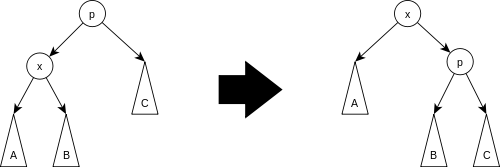Next, we consider the zig-zig operation, which happens when we traverse two edges in the same direction during the search. For example, if we traverse two "left" edges in the tree in a row, we will do a zig-zig operation. It looks as follows: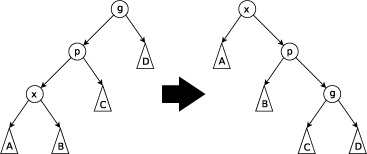Finally, there is the zig-zag operation, which is done when we traverse edges in two different direction, like "left" child and then the "right" child. The rotation goes as follows: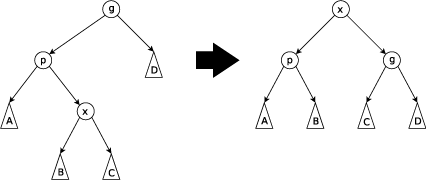An outline of the signature that we are going for is the following:

```      module type HEAP = sig
exception Empty_Heap
module M : ORDERED
type t
val create : unit -> t
val empty : t
val is_empty : t -> bool
val insert : M.t -> t -> t
val merge : t -> t -> t
val find_min : t -> M.t
val delete_min : t -> t
end
```
Your implementation of the functor needs to take in an `ORDERED` type and use the functions therein to implement all of the functions above. More specifically, you will have to implement the following signature
```      module type HEAPFUNCTOR =
functor (Elem : ORDERED) -> sig
exception Empty_Heap
module M :
sig
type t = Elem.t
val eq : t -> t -> bool
val lt : t -> t -> bool
val leq : t -> t -> bool
end
type t
val create : unit -> t
val empty : t
val is_empty : t -> bool
val insert : M.t -> t -> t
val merge : t -> t -> t
val find_min : t -> M.t
val delete_min : t -> t
end
```
We can break down the task as follows:
1. Decide on a good type to implement the backing data structure (`type t`), which must be a Splay Tree.
2. Use the splay tree to implement all of the above functions in the heap signature. (Hint: you might need to implement some hidden helper functions...)
To help you get started, we have included the file `bin_heap` which implements the Binomial Heap data type and the `HEAP` signature provided. You can use this to model your own solution.

To do: Turn in the solution in the file `splay_heap.ml`.

UPDATE: Here are the recommended (amortized) runtimes of the SplayHeap operations. Note that these runtimes will not be strictly enforced in the sense that as long as your solution does not time out in the test harness, it will be considered valid. This implies that all but the very slowest possible solutions will be ok. However, these runtimes are strongly preferred, and probably the most natural solutions to all of these problems.

Function Complexity
create O(1)
is_empty O(1)
insert O(log n)
merge O(n)
find_min O(log n)
delete_min O(log n)
Your code will not be tested with duplicates in the heap.

## Part 3 : Tries (20 points)

Several algorithms, including compression algorithms and string matching algorithms, make use of maps where the keys are sequences of values of a certain type. A trie or prefix tree is an efficient data structure for implementing the map ADT for this kind of map. The set of elements from which key sequences are built is called the alphabet of the trie.

A trie is a tree structure whose edges are labeled with the elements of the alphabet. Each node can have up to N children, where N is the size of the alphabet. Each node corresponds to the sequence of elements traversed on the path from the root to that node. The map binds a sequence s to a value v by storing the value v in the node corresponding to s. The advantage of this scheme is that it efficiently represents common prefixes, and lookups or inserts are achieved using quick searches starting from the root.

Suppose we want to build a map from lists of integers to strings. In this case, edges are labeled with integers, and strings are stored in (some of) the nodes. Here is an example: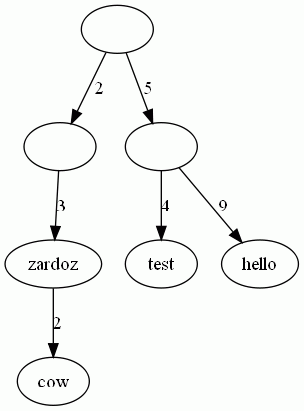The above trie describes the map ```{[2; 3]->"zardoz", [2; 3; 2]->"cow", [5; 4]->"test", [5; 9]->"hello"}```. The values are stored in the nodes. Note that not all nodes have values. For instance, the node that corresponds to the list `` has no value. Hence, `` is not in the map.

A cursor is a data structure that points to a specific node in a trie. It is useful to define a cursor pointing into a trie when performing lookups on lists of integers in order to advance through the trie number by number.

In this part you will implement tries that map lists of integers (`int list`) to values of arbitrary types (`'a`). We have provided you the following:

1. Interface specifications. Before you begin implementing the trie data structure, you should read over the type and representation of data in the signature `TRIE`.

2. Representation. We have given you a representaion that will help simplify the implementation of the functions below. Briefly inspect the functions below to understand the representation and how it can be applied to implement the desired functions.

1. Trie operations. Implement the `empty` value, representing an empty trie, and the functions `put`, `get`, `size`. The `put` function adds a new mapping to a Trie. If the value to be inserted is associated with a key list that is already bound to another value, then the code should replace this mapping by binding the key to the new value. The function `get` returns the value bound to the given key list in the trie. If the key does not exist in the trie, or if it exists in the trie but is not bound to any value, then an exception `NotFound` must be raised. Finally, the function `size` returns the number of mappings being stored in the map (note that this is not the same as the number of nodes).

```val empty: 'a trie
val put: 'a trie -> int list -> 'a -> 'a trie
val get: 'a trie -> int list -> 'a
val size: 'a trie -> int
```
2. Cursor support. Next, define an appropriate type to describe a cursor for a trie structure. Make sure the type contains enough information to support the cursor operations defined below.

```type 'a cursor = (* your implementation here *)
```

Implement the following functions:

```val cursor: 'a trie -> int list -> 'a cursor
val advance: 'a cursor -> int -> 'a cursor option
val getc: 'a cursor -> 'a option
```

The function `cursor` returns a cursor positioned at the node that corresponds to the int list given as an argument. If the list doesn't exist in the trie, the exception `NotFound` is raised. The function `advance` moves the cursor one position down the tree, following the edge that corresponds to the integer passed as an argument. This function returns `None` if no such edge exists. The function `getc` yields the value stored at the node that the cursor points to.

To do: Turn in the solution in the files `trie.mli` and `trie.ml`.

## Part 4 : The T9 Algorithm (25 points)

T9 is a technology used on many mobile phones to make typing text messages easier. The idea is simple - each number of the phone's keypad corresponds to 3-4 letters of the alphabet. To type a word like "TEST", you type the keys 8-3-7-8 corresponding to these letters. The phone then searches an internal dictionary for any possible words of the form {TUV}-{DEF}-{PQRS}-{TUV}, of which "TEST" is the most common (or only) word. Most phones use additional intelligence to improve performance of this basic algorithm. For example, many phones will notice when you type in a word that is not in its dictionary, and will add that word. Others keep track of the frequency of certain words and favor those words over other words that have the same sequence of keypresses.

Your task in this problem set is to implement a basic T9 algorithm. It will be particularly imporant in this exercise to choose data-structures that are well-suited to the following features that we are asking you to implement.

This T9 algorithm will have a word count associated with each of the words in its internal dictionary. When words are suggested, words that are used more often are suggested before rarer words that may fit the entered keystrokes, but are less common. Likewise, when a candidate word is selected, this internal word count must be increased.

The T9 module also behaves much like a state machine. At any point in time, it will have processed a certain set of keystrokes. If a new keystroke is entered by calling `enter_digit`, the returned T9 object should have its internal state updated to reflect this. Likewise, when `suggest_next_word` is called, the returned object should keep track of this fact and suggest a different word when this function is called a second time on the new T9 object.

In the file `t9.ml`, you will need to implement the following functions:

```(* update t9 w adds w to the T9 object if it does not exist, or increments
* the count by one if it does. *)
val update_word : t9 -> string -> t9

(* enter_digit t9 n attempts to return a T9 object in a new state, with
* the digit n typed.  If no words can be formed after adding this new digit,
* the input T9 object should be returned unchanged. *)
val enter_digit : t9 -> int -> t9

(* suggest_next_word t9 returns the next candidate word for completion at the
* current state of the input T9 word set, as well as a T9 object with the
* state updated to reflect this. *)
val suggest_next_word : t9 -> string option * t9

(* choose_word t9 confirms the selection of the current word, incrementing
* the frequency count and restarting the selection process *)
val choose_word : t9 -> t9
```

The specifications for these functions is given in the included .mli file, which you should not modify. We have provided an appropriate type for the T9 object. Be sure to think about about how to implement all of the above functionality given our choice of data structure, before you write too much code.

We will also provide you a file `dictionary.txt` that contains all of the words we want you to search for. We have also provided the file `dictionary.ml` in which the dictionary is read into a new T9 object.

The file I/O has been provided for you, but you should review how this works in OCaml. A nice tutorial on reading and writing files can be found here. You can also check out the OCaml documentation here. All of the provided files can be found on CMS.

Good luck and get started early! Please use Piazza or come to office hours if you have any questions.

To do: Turn in the solution in the file `t9.ml`.

UPDATE: Here are some additional clarifications that may remove any ambiguity in the specification:

1. The function `lookup_code` does not need to be implemented, however you may find it useful in implementing `update_word`. All that this function does is enter each digit in the code into the trie. This function will not be graded
2. If you call the function `choose_word` and there are no suggestions left, raise an error.
3. `choose_word` should return the word most recently suggested by `suggest_word`.
4. The cursor is reset to the root at every call to `update_word`
Here are some sample query sequences to test your trie on:
```let a = update_word empty "duck duck dual";;
let a = enter_digit a 3;;
let a = enter_digit a 8;;
let a = enter_digit a 2;;
let a = enter_digit a 5;;
let (w,a) = suggest_next_word a;; (* Some "duck" *)
let (w,a) = suggest_next_word a;; (* Some "dual" *)

let a = update_word empty "hello";;
let a = lookup_code a (convert_to_nums "hello");;
let (w,a) = suggest_next_word a;; (* Some "hello" *)

let a = update_word a "dual";;
let a = update_word a "duck";;
let a = update_word a "duck";;
let a = update_word a "duck";;
let a = update_word a "dual";; (* [("duck", 3); ("dual", 2)] *)

let a2 = enter_digit a 3;;
let a2 = enter_digit a2 8;;
let a2 = enter_digit a2 2;;
let a2 = enter_digit a2 5;;
suggest_next_word a2;; (* Some "duck" *)

let a = lookup_code a (convert_to_nums "eval");;
let (w,a) = suggest_next_word a;; (* Some "duck"*)
let (w,a) = suggest_next_word a;;(* Some "dual"*)
let a = choose_word a;;
let a = lookup_code a (convert_to_nums "EVAL");;

let (w,a) = suggest_next_word a;; (* Some "dual" *)
let (w,a) = suggest_next_word a;;(* Some "duck" *)
let (w,a) = suggest_next_word a;;(* Some "dual" *)
let a = choose_word a;;
let a = lookup_code a (convert_to_nums "dubl");;
let (w,a) = suggest_next_word a;; (* Some "dual" *)
let a = choose_word a;;

let a = enter_digit a 3;;
suggest_next_word a;; (* None *)

```

`sh build.sh`
```chmod +x build.sh
`build.bat`